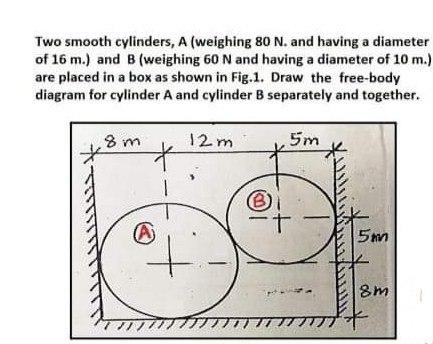# Two smooth cylinders, A (weighing 80 N. and having a diameter of 16 m.) and B (weighing 60 N and having a diameter of 10 m.) are placed in a box as shown in Fig. 1. Draw the free-body diagram for cylinder A and cylinder B separately and together.

Question-AnswerCategory: Engineering MechanicsTwo smooth cylinders, A (weighing 80 N. and having a diameter of 16 m.) and B (weighing 60 N and having a diameter of 10 m.) are placed in a box as shown in Fig. 1. Draw the free-body diagram for cylinder A and cylinder B separately and together.

Two smooth cylinders, A (weighing 80 N. and having a diameter of 16 m.) and B (weighing 60 N and having a diameter of 10 m.) are placed in a box as shown in Fig. 1. Draw the free-body diagram for cylinder A and cylinder B separately and together.